# How to Use the Excel REPLACE FunctionClaudia Buckley#### Join the Excel conversation on Slack

Ask a question or join the conversation for all things Excel on our Slack channel.

The Excel REPLACE function replaces a portion of text in a string by using a position number. No doubt you can think of several obvious use cases for this, but perhaps we can also give you a few not-so-obvious situations in which REPLACE might come in handy.

First, let’s learn the basic steps on how to use REPLACE in Excel.

## Syntax

The syntax of the REPLACE function is:

````=REPLACE(old_text, start_num, num_chars,new_text)`
```

Old_text is the full text string or cell reference containing the text to be replaced.

Start_num is the starting position number (or location) of the text string to be replaced.

Num_chars is the number of characters to be replaced

New_text is the new text which will replace the text to be removed

## Remarks

Whenever using explicit text values, they must be entered within double quotes. For example,

````=REPLACE(A1,1,2,“Microsoft”)`
```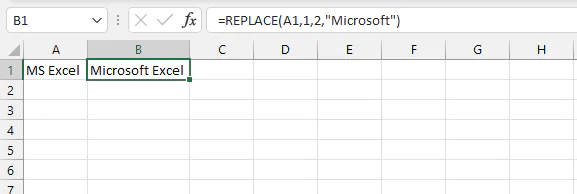• The REPLACE function does not alter the actual text in old_text. It returns the result of the replacement operation wherever the formula is entered.
• Num_chars cannot be a negative number.

## Using REPLACE with numeric values

1. The REPLACE function does not alter the actual text in old_text. It returns the result of the replacement operation wherever the formula is entered.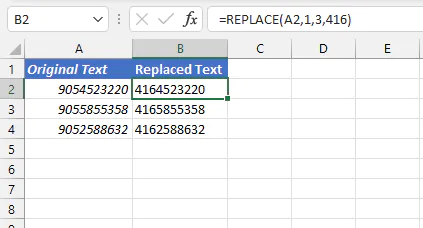2. Num_chars cannot be a negative number.
3. Your return value will be stored as text. REPLACE is a text function, so even though the return value may look like numbers, Excel has stored them as text. This means you won’t be able to perform mathematical calculations with them unless you change the number format after you get the result.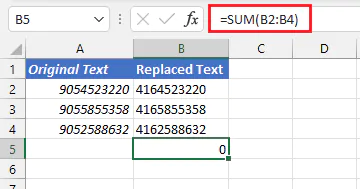## Using REPLACE with dates

Using the REPLACE function with dates means remembering that all dates in Excel are actually serial numbers. For example, the date displayed as December 31, 1999, is actually stored in Excel as the serial number 36525. We usually customize that number to display it as a date we recognize, but Excel only understands it in terms of its serial number.

It, therefore, means that working with dates usually requires special handling. Since REPLACE is one of Excel's "text" functions, we can apply the actual TEXT function to turn dates into text values and then perform the needed replacements.

The syntax of the TEXT function is

````=TEXT(value,format_text)`
```

Value is the numeric value to be converted into text. This can also be a cell reference that carries a numeric value.

Format_text is the text format that you would like to apply to the value. The format_text argument must be written in double quotation marks, for example:

``=TEXT(A1,"dd-mmm-yyyy")``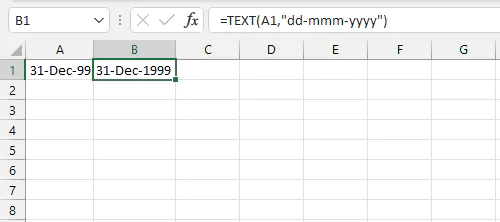The REPLACE function can now be used to make the changes we want. Instead of writing a brand new formula in another cell, we can simply use the TEXT formula as the old_text of the REPLACE function. To change the year to 2000, the following formula works.

````=REPLACE(TEXT(A1,"dd-mmm-yyyy"),8,4,2000)`
```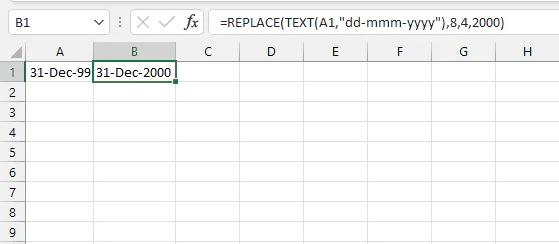## Insert characters into a text string

Did you ever think about using REPLACE to insert characters into a text string? Remember, the num_chars argument is not limited to values greater than 0!

With the following formula, we can insert a number at the beginning of the phone numbers by using 0 as the number of characters to be replaced.

``=REPLACE(A1,1,0,1)``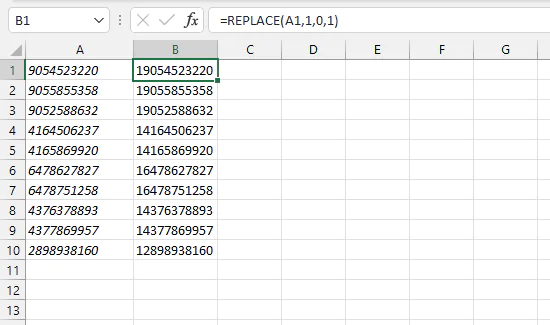With this little trick, Excel does not actually replace any of the existing characters in the original string. Note also that the new_text argument is not enclosed in double quotes this time because it is a numeric character.

## Remove characters from a text string

Similarly, we can remove certain characters from within a text string by using a pair of empty double quotes as our new_text argument.

````=REPLACE(A2,1,9,“”)`
```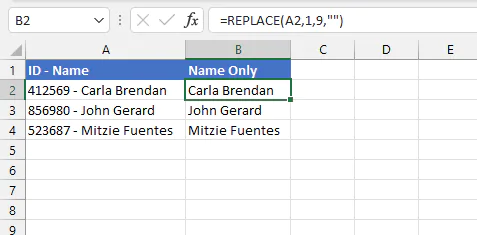Since the character position of the ID number is known, and there is a fixed number of digits, we can determine that replacing the first 9 characters with nothing (“”), will effectively remove those characters from the string.

## Replace a substring if present

The above examples assume that we know the position number of the text we want to replace. If we don’t know the position number, we can combine REPLACE with the SEARCH function. The syntax of the SEARCH function is:

``=SEARCH(find_text, within_text, [start_num])``

These arguments are explained as follows:

• Find_text is the text string or character you are searching for
• Within_text is the cell reference or text string where the character(s) is/are located
• Start_num (optional) is the position number of the character where the search should begin. If this argument is omitted, SEARCH starts looking from the first character of the string

Since the return value of the SEARCH function is a position number, and the REPLACE function needs a position number for its second argument, we can use the SEARCH formula as the second argument of the REPLACE function.

The formula below searches for the word “song” and replaces it with the word “poem” if it is present.

````=REPLACE(A2,SEARCH("song",A2),4,"poem")`
```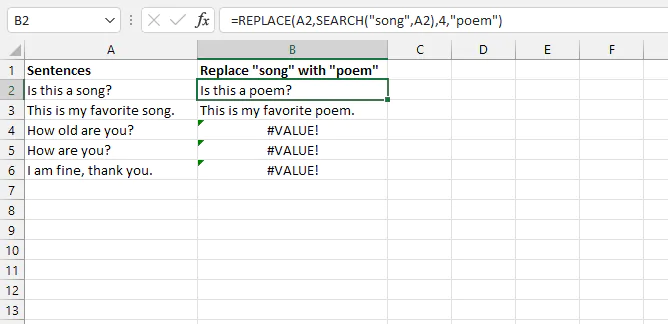Excel will calculate the inner formula first, so it searches for the text “song” in cell A2 and stores the position number (11) in the background. The result of the SEARCH formula is used in place of the start_num argument of the REPLACE function.

With the above formula, when text strings do not contain the substring searched for, Excel will return a #VALUE! error. This can be avoided by using the IFERROR function to return an alternative result. The syntax of the IFERROR function is:

````=IFERROR(value, value_if_error)`
```

By using the REPLACE/SEARCH formula combination in place of the value argument of the IFERROR function, we can alter the contents of the cells containing the values we searched for while leaving the others unchanged.

``=IFERROR(REPLACE(A2,SEARCH("song",A2),4,"poem"),A2)``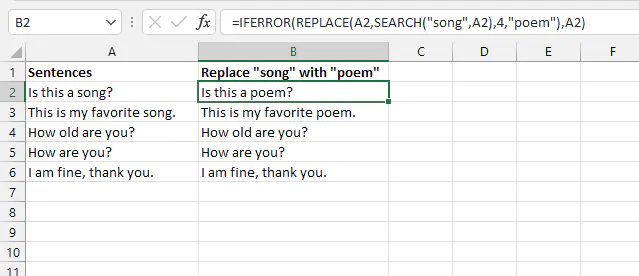In the formula above, the last argument of the IFERROR function is set to return the original text if no match was found by the SEARCH argument.

Note: SEARCH and FIND are almost identical, the main difference being that FIND is case-sensitive, and SEARCH supports the use of wildcard characters.

## Other ways to replace words

There are other options for replacing text in Excel, including the SUBSTITUTE function, the CHOOSE function, and the Find and Replace command.

## REPLACE vs. SUBSTITUTE

One main difference between the REPLACE function and the SUBSTITUTE function is that REPLACE requires the position number of the text being replaced, while SUBSTITUTE looks for the actual text string, even if the position is unknown. From this explanation, it may seem that SUBSTITUTE is superior to REPLACE, but not necessarily.

SUBSTITUTE is quite useful when you know the actual text being replaced, but as we have seen earlier, that may not always be the case.

Below, we know the number of characters to be replaced, and we know where they are located. But since the exact text of the substring is unknown, the SUBSTITUTE function would not be very helpful here.

````=REPLACE(A2,1,6,B2)`
```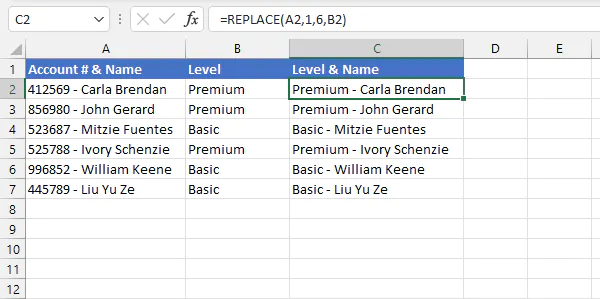Note above that the new_text was not placed within double quotes because we are replacing old_text with the value in cell B2, not with the text “B2”. The SUBSTITUTE function is more useful in the following instance, where the exact value of the text to be replaced is known. The syntax of the SUBSTITUTE function is:

``=SUBSTITUTE(text, old_text, new_text, [instance_num])``

If the last argument is omitted, SUBSTITUTE replaces all occurrences of the text being replaced.

``=SUBSTITUTE(A1,“2016”,“365”)``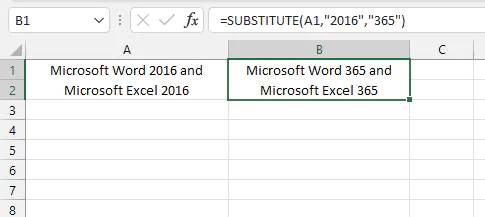Another key difference is that by using the position number, REPLACE allows you to specify the location of the character to be replaced, while SUBSTITUTE refers to the particular instance(s) of the text occurrence to be replaced.

## REPLACE vs. CHOOSE

The CHOOSE function uses a list of values as an index to determine which value should replace a given number (from 1-254).

The syntax of the CHOOSE function is:

````=CHOOSE(index_num, value1, [value2, value3],...)`
```

Index_num is the nth value to be returned. For example, an index_num of 1 will return the argument stated as value1. An index_num of 6 will return the argument stated as value6, and so on.

An example of how it works is shown below.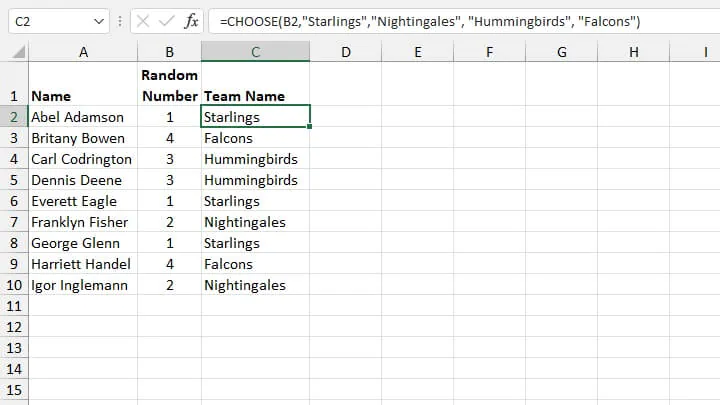Whether you use REPLACE, SUBSTITUTE, or CHOOSE all depends on what information is known and on what is needed at that time.

### Find and Replace command

To use the Find and Replace feature, choose Find & Select from the Home tab and click Replace. Or you can use the Ctrl+H shortcut key.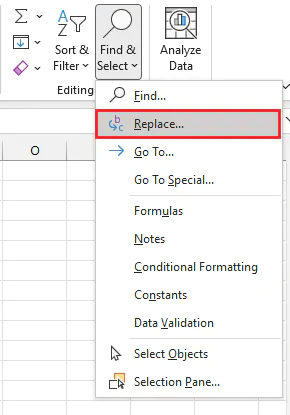From the Find and Replace window, input the text being replaced in the Find what: field, and enter the new text in the Replace with: field.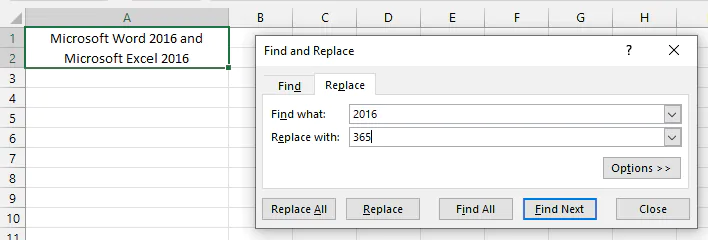To replace all occurrences of the old text, click Replace All. To replace a specific instance of the old text, click Find Next until Excel locates that occurrence, then clicks Replace.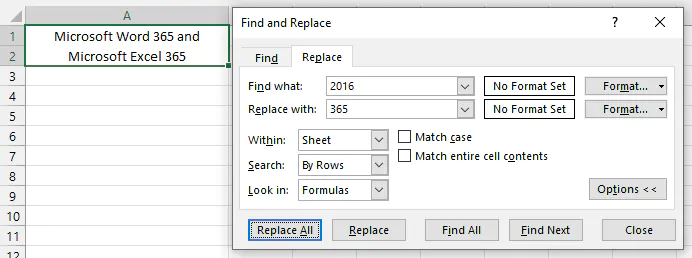Note that with the Find and Replace command, the original text itself is altered.

## Which method is best?

Each of the above methods of replacing text in Excel has its own benefit. Download the accompanying Excel workbook to get comfortable with using them all.

Ready to become a certified Excel ninja?